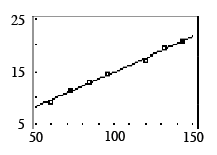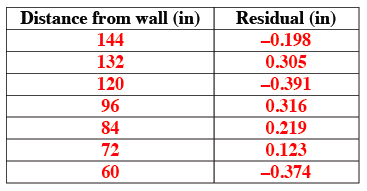### Home > GB8I > Chapter 4 Unit 5 > Lesson INT1: 4.2.1 > Problem4-62

4-62.

Robbie’s class collected the following view tube data in problem 4-1. Homework Help ✎

 Distance from wall (in) Width of field of view (in) $144$ $20.7$ $132$ $19.6$ $120$ $17.3$ $96$ $14.8$ $84$ $13.1$ $72$ $11.4$ $60$ $9.3$ checksum $\it 708$ checksum $\it 106.2$
1. Use your calculator to make a scatterplot and graph the least squares regression line (LSRL). Sketch the graph and LSRL on your paper. Remember to put a scale on the $x$axis and $y$axis of your sketch. Write the equation of the LSRL rounded to four decimal places.

See scatterplot below. $y = 1.6568 + 0.1336x$2. Using your calculator, determine the residuals. Make a table with the distance from wall (inches) as the first column and residual (inches) in the second column. What is the sum of the squares of the residuals?

• See table below.
Use your calculator to find the sum of the squares of the residuals.